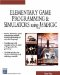# List of Figures

## Chapter 1: Initial Topics

Figure 1.1: Flight simulator screen shot.
Figure 1.2: Ship simulator screen shot.

## Chapter 2: 3D Game Basics

Figure 2.1: The one-dimensional world.
Figure 2.2: A two-dimensional world.
Figure 2.3: A 3D world.
Figure 2.4: An isometric view of a cube in a 3D world.
Figure 2.5: Positive and negative axes in the 3D world.
Figure 2.6: Triangle in 3D world.
Figure 2.7: 3D cube seen from the front.
Figure 2.8: Triangle in 3D world.
Figure 2.9: The image we wish to apply to triangle, with u,v coordinates marked .
Figure 2.10: Triangle with image mapped using u,v coordinates.

## Chapter 3: Your First Program

Figure 3.1: Initial Jamagic screen.
Figure 3.2: Jamagic project screen.
Figure 3.3: Jamagic project screen.
Figure 3.4: Getting back to your program.
Figure 3.5: The triangle we are constructing with three posts in a 3D world.
Figure 3.6: Jamagic default camera locationtoward the positive z-axis.
Figure 3.7: Starting the Jamagic picture editor.
Figure 3.8: The Jamagic picture editor.
Figure 3.9: The image painted with the Jamagic picture editor.
Figure 3.10: Renaming the picture.
Figure 3.11: Image mapped on triangle.
Figure 3.12: Rectangle made of two triangles .
Figure 3.13: The image mapped onto the rectangle.
Figure 3.14: Adding a third triangle to our basic rectangle.

## Chapter 4: Simple Games

Figure 4.1: Screenshot of the Sphere1 project.
Figure 4.2: Rotation about the three axes.
Figure 4.3: Screenshot of the Sphere2 program for experimenting with shading techniques.
Figure 4.4: Sphere3 screenshot. Collision detection activated. The use of functions introduced.
Figure 4.5: Sphere4 project using fog and planes.
Figure 4.6: Sphere5 screenshot. Sounds and music have been added, and distances are computed and displayed.
Figure 4.7: Screen coordinate system.
Figure 4.8: Screenshot of Sphere6 game. The sphere must be maneuvered onto the landing surface before the sphere falls too far.
Figure 4.9: The Pythagorean theorem for finding the distance C between two points.
Figure 4.10: Distance between points in 3D space.
Figure 4.11: Screenshot of Sphere7 game.
Figure 4.12: Code to be commented out to prevent default window from being created.
Figure 4.13: Screenshot of Sphere8 game.
Figure 4.14: MilkShape 3D initial screen.
Figure 4.15: Points added to make island.
Figure 4.16: Points showing outline of island from top.
Figure 4.17: Points joined to make triangles.
Figure 4.18: You will be prompted for the directory where textures are located.
Figure 4.19: The imported files should be visible under the 3D category.
Figure 4.21: Screenshot from Sphere9. The sphere is replaced with a spaceship imported from the CD-ROM.
Figure 4.22: Texture pictures loaded with fighter.
Figure 4.23: Jamagic sound editor.
Figure 4.24: Zoom Feature of Jamagic sound editor.

## Chapter 5: Flight Simulators

Figure 5.1: Aircraft rotation about 3 axes: pitch, yaw and roll.
Figure 5.2: When an aircraft rolls to one side, it also turns to one side lift force points to the side as well as up.
Figure 5.3: The unit circle.
Figure 5.4: 0.524 radians (30 degrees) on the unit circle. Cosine is 0.867 and sine is 0.5.
Figure 5.5   Jet seen from the front in level flight. The z-axis points into the viewer. The x-axis runs horizontally. They y-axis runs vertically. Sine is 0.
Figure 5.6   Jet is rotated 0.196 radians about the z-axis. Sine is 0.195.
Figure 5.7   Jet is rotated 0.983 radians about the z-axis. Sine is 0.83.
Figure 5.8   Jet is rotated 1.57 radians about the z-axis. Sine is 1.0.
Figure 5.9   Jet is rotated 3.148 radians about the z-axis. Sine is 0.
Figure 5.10   Jet is rotated 4.72 radians about the z-axis. Sine is 1.
Figure 5.13: Jet pitching up. Airspeed goes down.
Figure 5.14: An aircraft moves 500 miles in one hour and climbs 6 miles.
Figure 5.15: The sine of the aircraft angle times its airspeed is the speed in the vertical direction (rate of climb).
Figure 5.16: The jet with a focus value of 10.
Figure 5.17: The jet with a focus value of 50.
Figure 5.18: The aircraft heads north. The runways run perpendicular east-west.
Figure 5.19: Compass directions and corresponding degree headings.
Figure 5.20: The final flight simulator with analog type instruments. The compass heading in particular is useful in landing the jet properly.
Figure 5.21: The gauge background sprite. Note that the rectangular area is left as the original white color , which is transparent to Jamagic . Also note the hot-spot location at the center of the gauge and the hot-spot icon beneath the text icon.
Figure 5.22: The indicator dial. Note that the hot spot is at the bottom.
Figure 5.23: The compass gauge with compass directions in bold letters .

## Chapter 6: Ship Simulators

Figure 6.1: Ship simulator screenshot.
Figure 6.2: The instrument panel. The throttle indicator is at the right and the bow thruster is near the center.
Figure 6.3: ShipSim2 screenshot. The north side of main island.
Figure 6.4: Overhead view of the game scene. The dock is on the north side of the island.Elementary Game Programming & Simulations Using Jamagic (Charles River Media Game Development)
ISBN: 1584502614
EAN: 2147483647
Year: 2002
Pages: 105
Authors: Sergio Perez